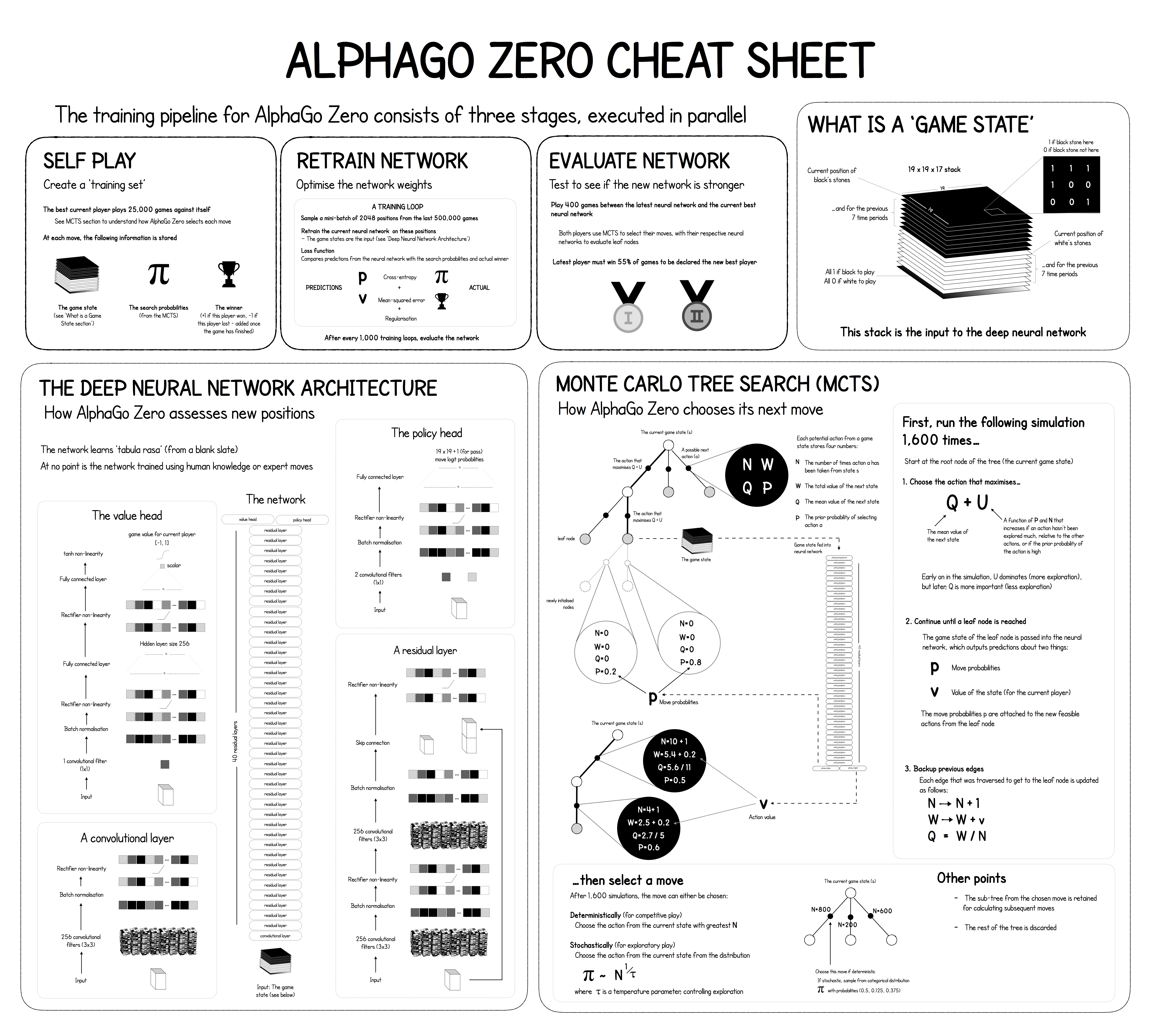# Advanced Introduction to C++, Scientific Computing and Machine Learning

Claudius Gros, SS 2023

Institut for Theoretical Physics
Goethe-University Frankfurt a.M.

# simple vs. complex problems### simple problems

• limited number of variables
• exact solution of interest, like for XOR
: lowest energy state
: gradient descent does not work
backpropagation fails for simple problems

### complex problems

• large numbers of variables
• many 'good' local minima are ok
• humans would not survive, if it was otherwise
given enough (labeled) training data, large
classes of complex problems are 'solvable'
• like playing Go, recognizing a face, ...
• counter-examples: cryptology, ...

# deep networkspruning
 removing : weak links; $|w_{ij}|$ well below average reduces : network complexity; overfitting

data preprocessing
 whitening : covariance matrix $\to$ identity matrix : all data equally relevant
• a large number of hidden layes
allows for highly non-linear and
hierarchical classification
• huge number of parameters and options,
for training and networks design
• learing rate $\to$ adaptive control
• initialization of weights
• ...
• 'shallow nets' have not too many hidden layers

### batch learning

• synaptic weights adjusted after presenting a 'batch'
of $N_d\$ training data $\ \ (\mathbf{I}_\alpha,\mathbf{y}_\alpha)$

$E = \frac{1}{2 N_d } \sum_\alpha \big|\mathbf{y}_\alpha-\mathbf{y}\big|^2, \qquad\quad \mathbf{y}=\mathbf{y}(\mathbf{I}_\alpha)$

### 'online' learning

• ML
:training on a pair by pair basis
• neurosciences
: if the cognitive system is continuously active
: no distinct phases for learing and performing

### offline learning

• distinct modes for trainging and predicting
: most ML algorithms are offline (but not the brain)

# deep belief nets (DBN)### stacked RBMs

• data on input layer
train
input layer $\ \leftrightarrow\$ first hidden layer
• first hidden layer acts as data
train
first hidden layer $\ \leftrightarrow\$ second hidden layer
• repeat
 $\to$ hierarchical feature representation by hidden nodes

### data availability

• large amount of unlabelled data
images from web, ...
• much smaller amount of labelled data

### semi-supervised learning

• unsupervised pre-training followed by supervised learning
train a net of stacked RBMs with unlabelled data
add a final output node connected to top hidden layer
use backpropagation on labelled data
to fine-tune connection weights
• graph undirected, besides links to output layer

# autoencoder### dimensionality reduction

$\fbox{$\phantom{\big|}$input$\phantom{\big|}$} \quad\equiv\quad \fbox{$\phantom{\big|}$output$\phantom{\big|}$}$

• hidden layer with low numbers of units
$\to\$ information bottleneck
autoencoders generate low-dimensional
representations of the (raw) data;
in the 'latent space'
• training via backpropagation of errors

### denoising

• using on-purpose corrupted input data
forces the autencoder to learn to
reconstruct the orginal data

### stacked autoencoders

• two meanings
• several hidden layers
• stacked to form a deep network
• stacking for deep networks
: bottleneck layer of one encoder servers as
: the data layer of the subsequent encoder

# deep learning building blocksautoencoder restricted Boltzmann machine recurrent network convolution network feedforward undirected recurrent hierarchical feedforward

### backpropagation through time

• recurrent networks $$\mathbf{y}(t+1) = \sigma\big(\hat{w}\cdot\underbrace{\mathbf{y}(t)}_{\textstyle=\, \sigma\big(\hat{w}\cdot \underbrace{\mathbf{y}(t-1)}_{\textstyle=\,\sigma(\hat{w}\cdot \underbrace{\mathbf{y}(t-2)}_{\textstyle\dots} -\mathbf{b})\hspace{-10cm}} -\mathbf{b}\big)\hspace{-12cm}}-\mathbf{b}\big)$$ may be viewed as layered feedforward (in time) networks
$$\fbox{\phantom{\big|} \mathbf{y}(t+1) \phantom{\big|}} \quad\leftarrow\quad \fbox{\phantom{\big|} \mathbf{y}(t) \phantom{\big|}} \quad\leftarrow\quad \fbox{\phantom{\big|} \mathbf{y}(t-1) \phantom{\big|}} \quad\leftarrow\quad \fbox{\phantom{\big|} \mathbf{y}(t-2) \phantom{\big|}} \quad\leftarrow\quad\dots$$
• backpropgation algorithm applicable
• select time horizon
• identical inter-layer weight matrices

# receptive fields as convolutions• convolution

$\qquad\quad (f *g)(x) = \int_{-\infty}^\infty f(x-y)\, g(y) \, dy$

or

$\qquad\quad (w *y)_i = \sum_j w_{i-j}\, y_j$
• kernel $\ \ w_{ij} \to w_{i-j}$### receptive fields

• retina/visual cortex
• center on/off
• edge/direction/contrast detection
• gratings
• color
• ...

### convolution scanning of 2D data

• raster image for a given kernel# convolution networks

• identical objects may look very different
• location
• visual size
• distortions
• illumination effects
• ..

### convolution nets

• specialized to deal with invariances
: translation, scaling, skewing, distortion, ...
extended set of kernels
$\qquad\Rightarrow\qquad$
rastering
$\qquad\Rightarrow\qquad$
data convolution
• feature $\ \hat{=} \$ kernel
• topographic dimensionality reduction
: $w_{ij}\to w_{i-j}\$ sparse network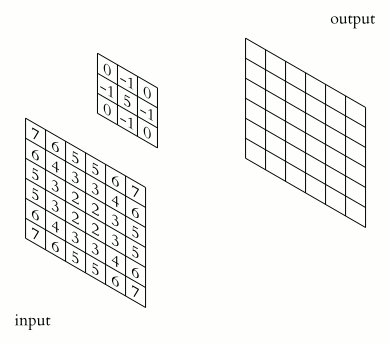### pooling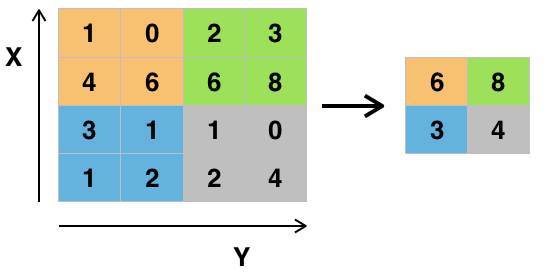$\qquad$ convolution $\ \to \$ feature map pooling : subsampling : dimensionality reduction : e.g. max-pooling

### what makes it work

• kernels:
: reduced number of weights
: translational invariance
• pooling
: non-linearity
: dimensionality reduction
• training via backpropagation
: max-pooling weights $\ 0/1$
: kernels optimized for each layer
• ReLu neuron (rectified linear units)
: scale invariance
: computational efficiency
: error (back-) propatation# convolution net - illustration

• Visualizing and understanding convolutional networks,
M.D. Zeiler and R. Fergus,
European Conference on Computer Vision. Springer 2014.
• 1.3M/50k/100k training/validation/test data (parameters/hyper-parameters/performance)
$256 \times 256$ images (down-sampled, RGB mean zero)
10 sub-crops of size $254\times 254$ per image (center/corners/flip)
1000 label categories
• 5 convolution layers, 3 fully connected (4096 neurons)
a single pooling layer (first $\to$ second)
96 kernels of size $11 \times 11 \times 3$ (x,y, color); stride 4 (first layer)
256 kernels of size $5 \times 5 \times 48$ (second layer)
384 kernels of size $3 \times 3 \times 256$ (third layer)
384 kernels of size $3 \times 3 \times 192$ (forth layer)
256 kernels of size $3 \times 3 \times 192$ fifth layer)
• 60 million parameters; batch size 128; 70 epochs; 12 days, one GPU
• inversion deconvolution net
: map feature activites back to input pixels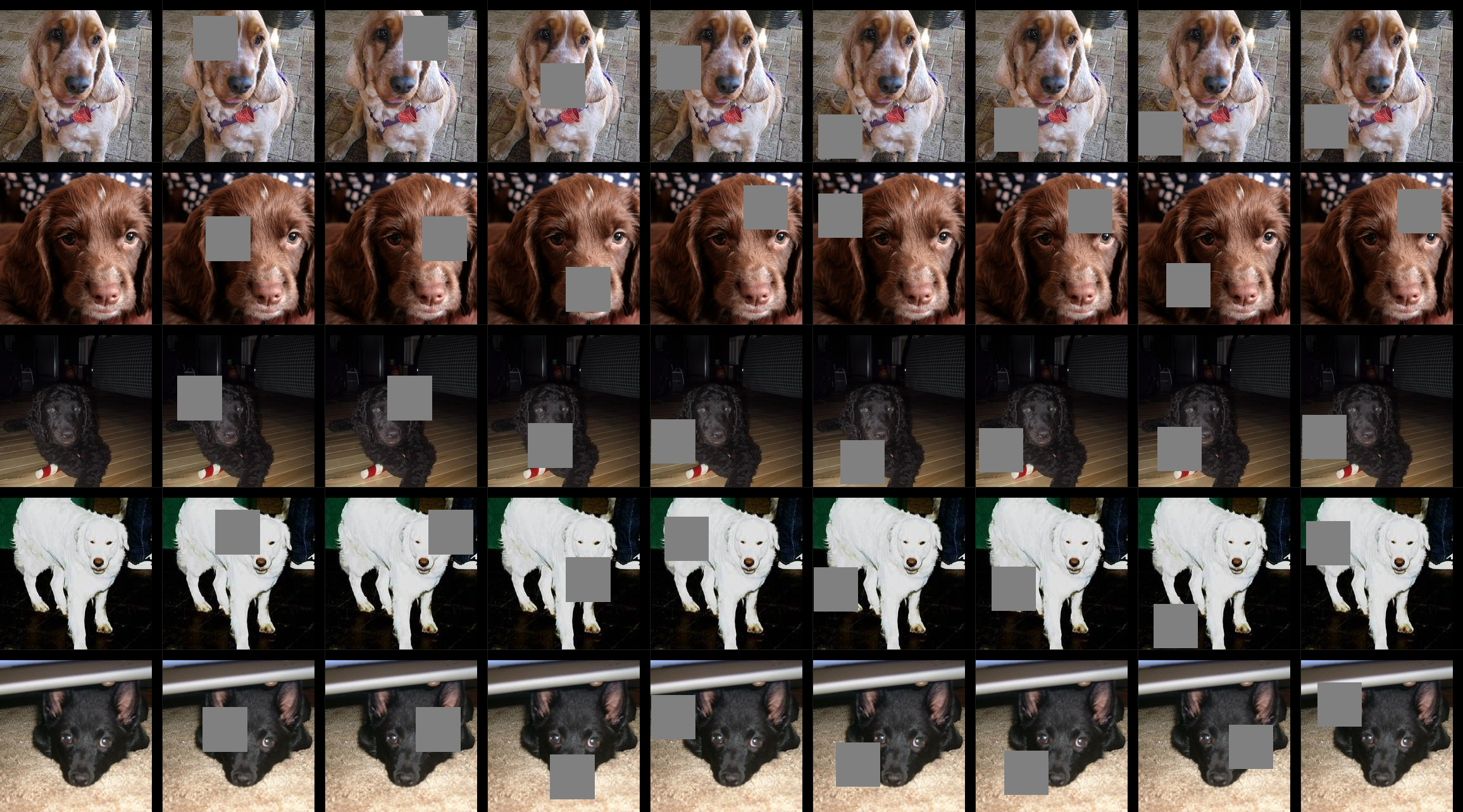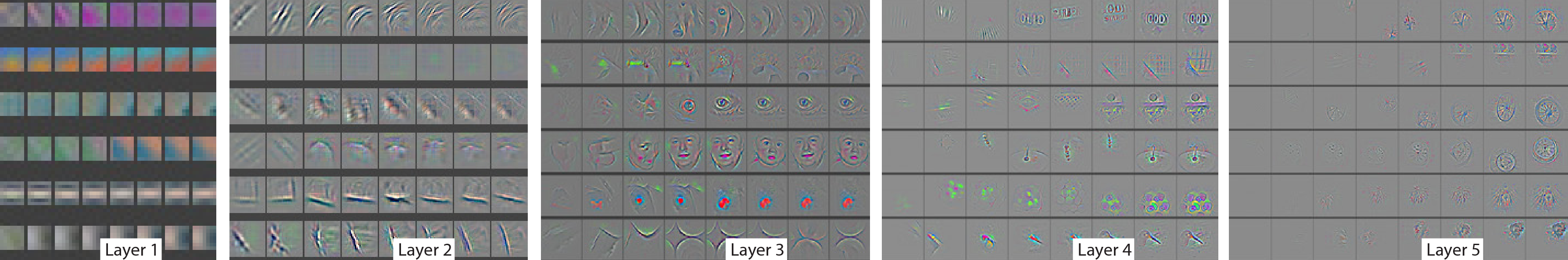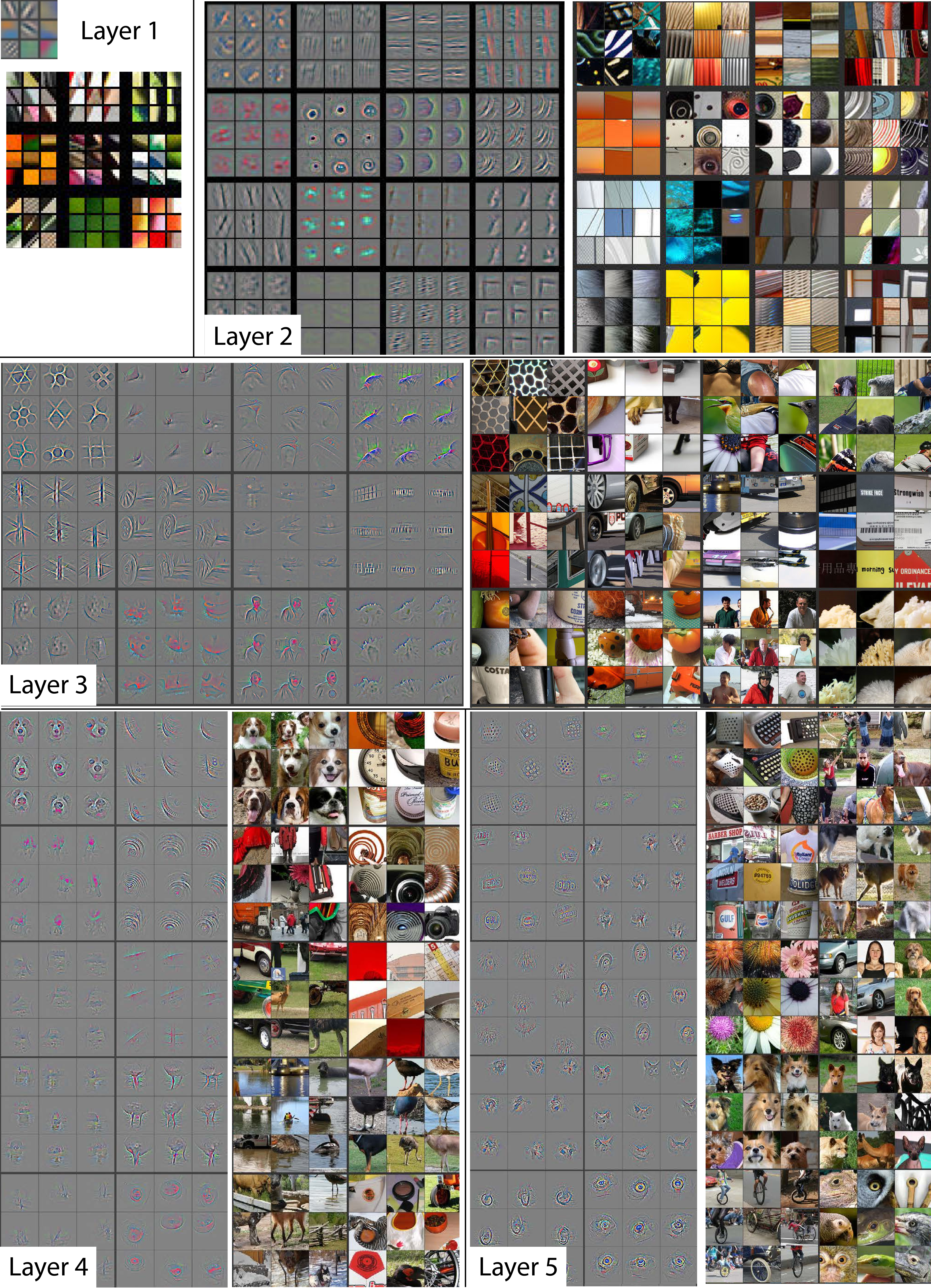# fooling deep networks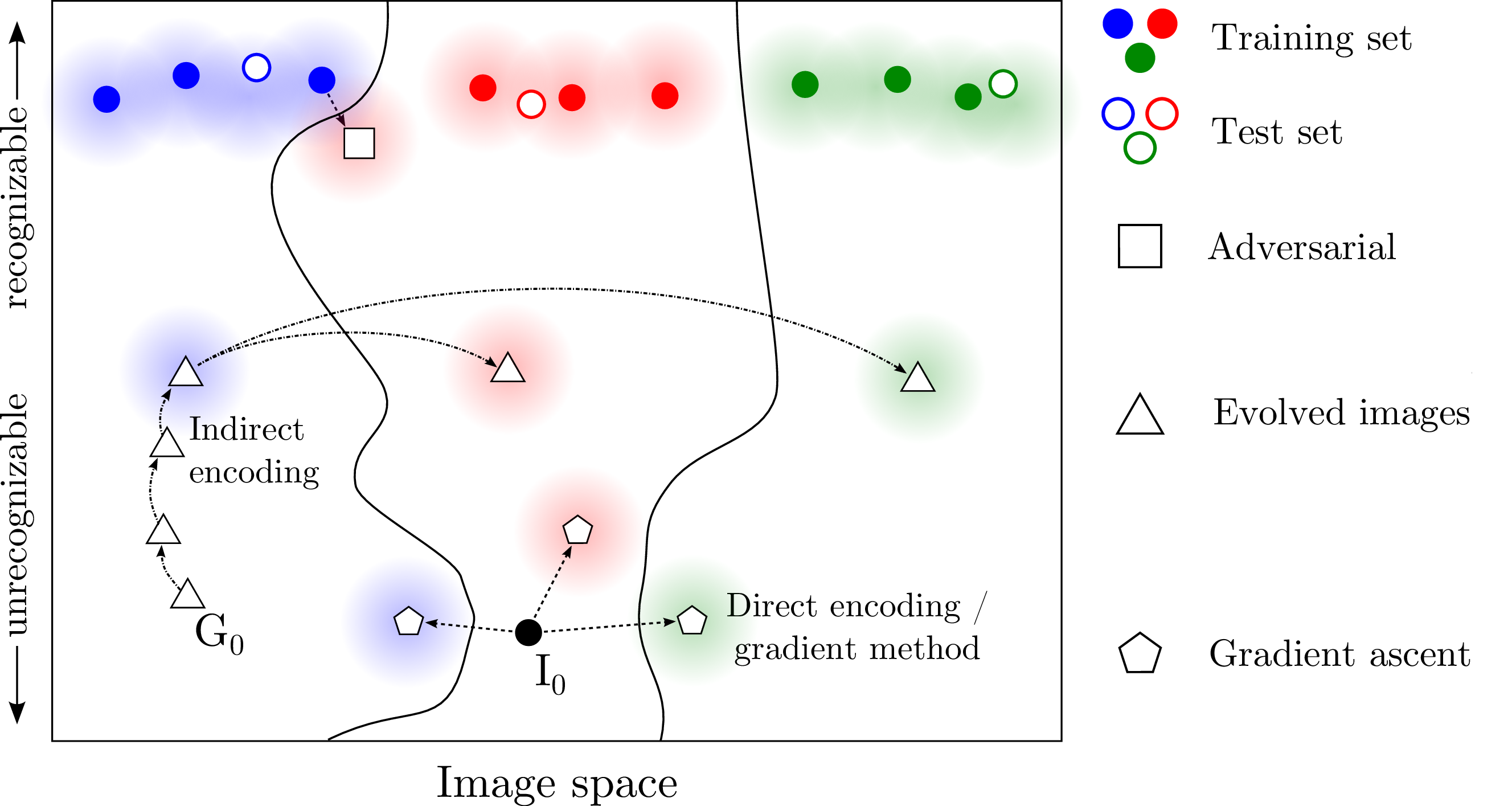• evolved recognizable images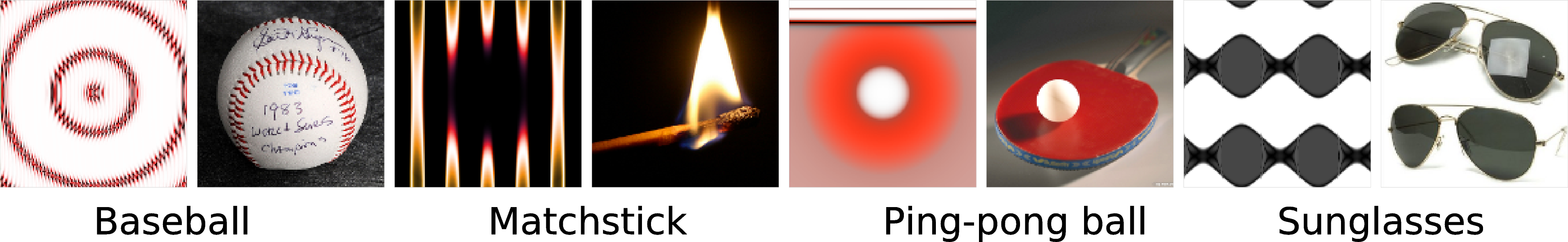• evolved unrecognizable images, mean confidence 99.12%
$\to \$ implications for "human-level performance"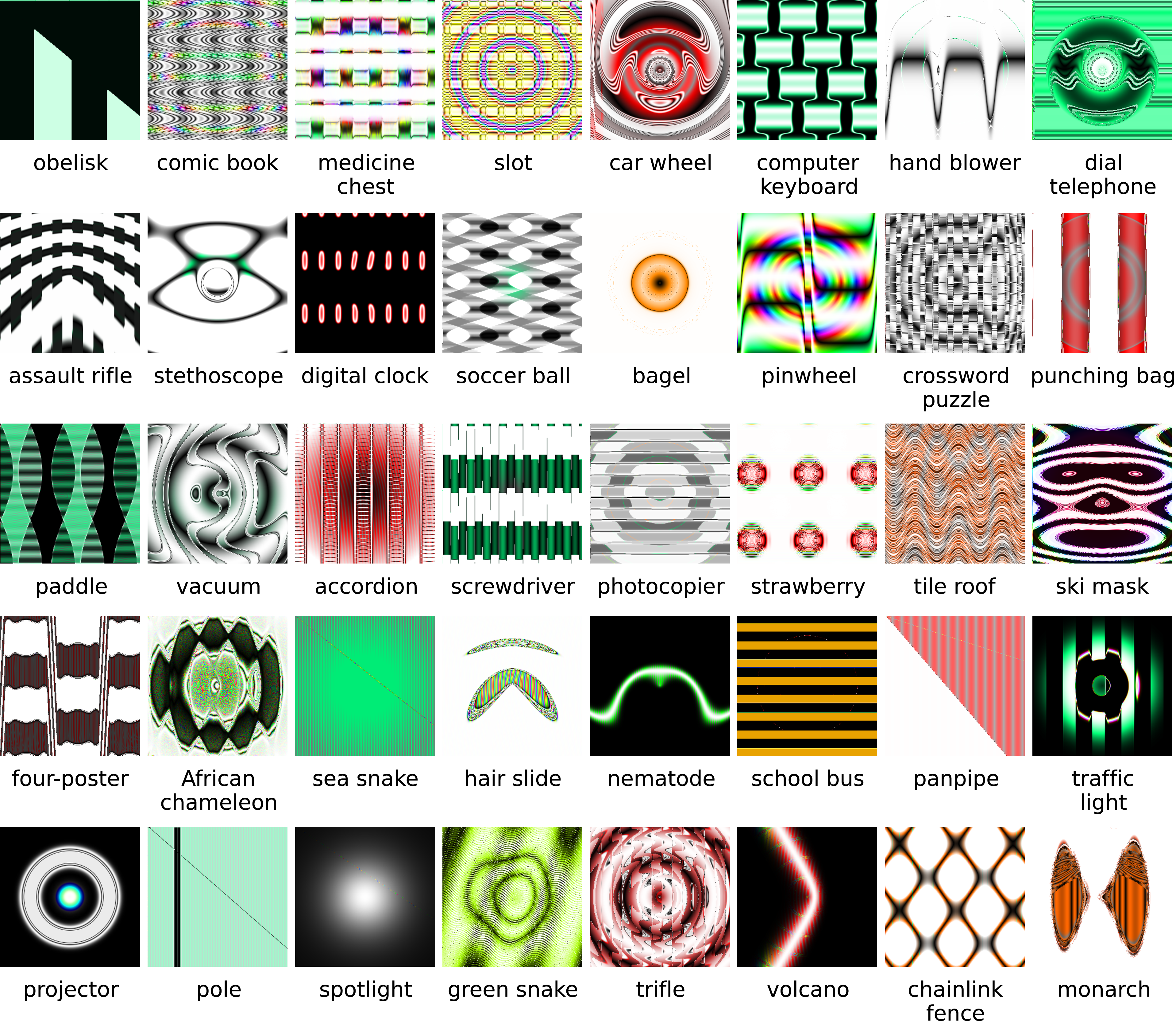+ 0.007 x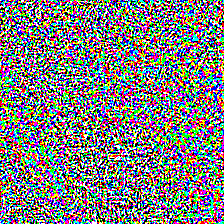=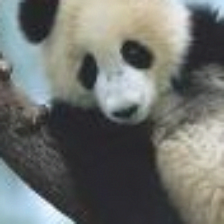"panda" "nematode" "gibbon" 57.7% confidence 8.2% confidence 99.3 % confidence

### performance / confidence

• performante $\ \hat{=} \$ average success on test data
• confidence for a single prediction
: a range of methods
• most simple:
• diregard activities below a certain confidence threshold
• normalize activity of the remaining output neurons to unity
• confidence/class as the activity of most active output neuron

# attacking deep networks

 original tempered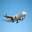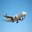### image datasets

• CIFAR-10: 60000 32x32 color images in 10 different classes
• SVHN: Street View House Numbers (SVHN) Dataset

### temper train data

• one random bit: RGB-blue set to zero
$\hat{=}\$ camera with a technical defect
• training with tempered $\ \Leftrightarrow\$ testing with un-tempered data

### missclassification induced by training data tempering

• percentage miss-classifaction
• selection of network architectures
$$\begin{array}{lcccc} \hline & \rlap{\text{baseline}} & & \rlap{\text{tampered}} \\ & \text{CIFAR} & \text{SVHN} & \text{CIFAR} & \text{SVHN} \\ \hline \text{optimal case} & 0 & 0 & 100 & 100 \\ \hline \text{BCNN} & 28.7 & 12.9 & 87.2 & 91.4 \\ \text{AlexNet} & 11.1 & 5.5 & 83.7 & 97 \\ \text{VGG-16} & 5.3 & 3.7 & 90.1 & 98.9 \\ \text{ResNet-18} & 23.8 & 3.6 & 42.4 & 40.9 \\ \text{SIRRN} & 4.7 & 3.9 & 74.1 & 89.5 \\ \text{DenseNet-121} & 2.6 & 2.6 & 60.7 & 68.1 \\ \hline \end{array}$$

# PyTorch

• PyTorch is an open source machine learning framework
:: written in Python; C++; CUDA
:: supports CPU, GPU (with CUDA)
• other Python ML frameworks
:: Keras (within TensorFlow)
:: Pandas
• loop-free computational graphs graphs
• edges: matrices of data (tensors, if higher-dimensional)
• vertices: machine-learning operations
$\color{red}\Rightarrow\$ automatic gradient evaluation
#!/usr/bin/env python3

import torch                     # PyTorch needs to be installed

dim = 2
eps = 0.1
x = torch.ones(dim, requires_grad=True)  # leaf of computational graph
print("x      : ",x)
print("x      : ",x.data)

y = x + 2
out = torch.dot(y,y)             # scalar product
print("y      : ",y)
print("out    : ",out)
print()

out.backward()                   # backward pass --> gradients

with torch.no_grad():            # detach from computational graph
x -= eps*x.grad                # updating parameter tensor

print("x      : ",x.data)
torch.dot(x+2,x+2).backward()


# AlphaGo zero### game of Go

• number of configurations
$3^{19\cdot19} \approx 1.7\cdot10^{172}$
• 2017 AlphaGo zero (Goggle DeepMind)
: self-play ($5\cdot10^6$ games)
• deep neural net generates estimates for
• value of a new configuration: probability to win
• policy to explore an yet untested move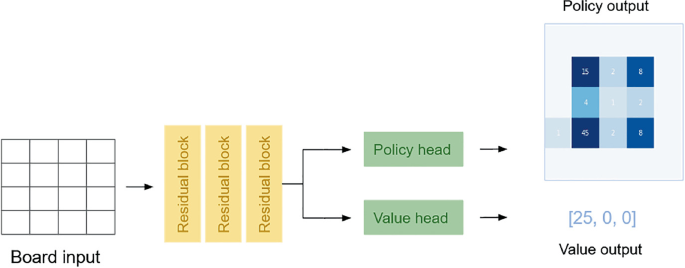• Monte Carlo tree search uses combination of
• exploitation maximize probability to win (value)
• exploration trying out something new (policy)
• 1600 Monte Carlo tree search steps
• next move selected
most explored configuration
• not: configuration most likely to win!

# Alphago zero cheat sheet